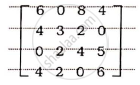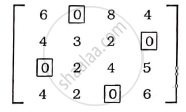# A job production unit has four jobs A, B, C, D which can be manufactured on each of the four machines P, Q, R and S. The processing cost of each job is given in the following table - Mathematics and Statistics

Sum

A job production unit has four jobs A, B, C, D which can be manufactured on each of the four machines P, Q, R and S. The processing cost of each job is given in the following table:

 Jobs Machines P Q R S Processing Cost (Rs.) A 31 25 33 29 B 25 24 23 21 C 19 21 23 24 D 38 36 34 40

How should the jobs be assigned to the four machines so that the total processing cost is minimum?

#### Solution

We can express the matrix form

[(31,25,33,29), (25,24,23,21), (19,21,23,24), (38,36,34,40)]

Subtracting the smallest element in each row from every element of it,

[(6,0,8,4), (4,3,2,0), (0,2,4,5), (4,2,0,6)]

Subtracting the smallest element In each column from very element of it.

[(6,0,8,4), (4,3,2,0), (0,2,4,5), (4,2,0,6)]

All the zeros of the above matrix are covered with minimum number of lines as below :No. of lines = No. of rows/columnsAssignment of jobs :

A → Q, B → S, C → P, D → R

Minimum cost = 25 + 21 + 19 + 34

= Rs 99

Concept: Assignment Problem
Is there an error in this question or solution?
2014-2015 (March)

Share